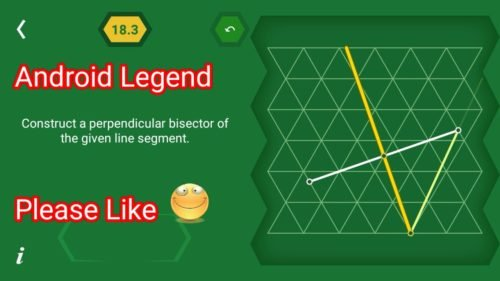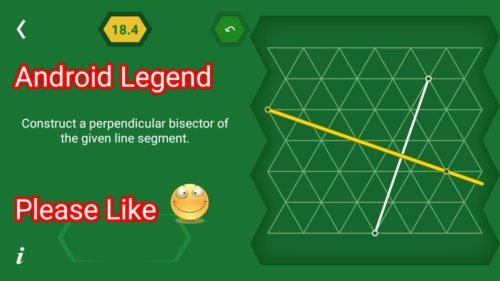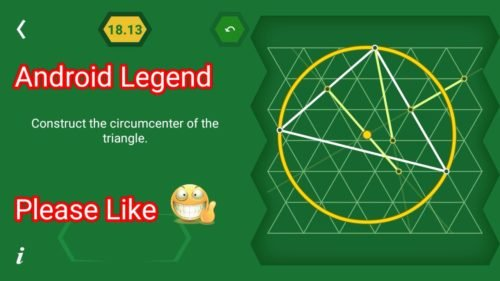# Pythagorea 60 degree Level 18.1 18.2 18.3 18.4 18.5 18.6 18.7 18.8 18.9 18.10 18.11 18.12 18.13 18.14 Solutions/AnswersPythagorea 60 degrees is android/iOS app developed by Horis International Limited. This game is mostly focused on geometric puzzles and construction. The workspace is divided into triangular grids lines aligned 60° to each other, that’s why this game names as pythagorea 60°. You should know all the basic Math operations. All lines and shapes are drawn on a grid whose cells are equilateral triangles. Most of the game levels can be answered using natural intuition and bye some basic laws of geometry.

You have to connect points on the grid using straight lines to construct an element, you can even use intersection points to draw. some levels are very easy some are of medium difficulty and some are very hard to solve, To help you, I am providing solutions to all the problems.

If you are here for levels other than ‘Perpendicular Bisectors’ Go to directory of all other levels at : http://www.puzzlegamemaster.com/pythagorea-60-complete/

• Pythagorea 60° Level 18.1: Construct a perpendicular bisector of the given line segment.• Pythagorea 60° Level 18.2: Construct a perpendicular bisector of the given line segment.• Pythagorea 60° Level 18.3: Construct a perpendicular bisector of the given line segment.• Pythagorea 60° Level 18.4: Construct a perpendicular bisector of the given line segment.• Pythagorea 60° Level 18.5: Construct the circumcenter of the triangle.• Pythagorea 60° Level 18.6: Construct the circumcenter of the triangle.• Pythagorea 60° Level 18.7: Construct a perpendicular bisector of the given line segment.• Pythagorea 60° Level 18.8: Construct a perpendicular bisector of the given line segment.• Pythagorea 60° Level 18.9: Construct the circumcenter of the triangle.• Pythagorea 60° Level 18.10: Construct a perpendicular bisector of the given line segment.• Pythagorea 60° Level 18.11: Construct a perpendicular bisector of the given line segment.• Pythagorea 60° Level 18.12: Construct the circumcenter of the triangle.• Pythagorea 60° Level 18.13: Construct the circumcenter of the triangle.• Pythagorea 60° Level 18.14: Construct the circumcenter of the triangle.Feel free to comment below if you have any doubts regarding the solutions. I will try to help you guys. All other levels are posted on this blog please visit them too.